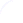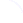Search
 Submission Procedure share: |Follow usavailable in:   PDF (128 kB) PS (134 kB)

get:
 Similar Docs BibTeX Write a comment
get:
 Links into Future

DOI:   10.3217/jucs-008-02-0270

### Synchronization and Stability of Finite Automata

Jarkko Kari (Department of Mathematics and Turku Centere for Computer Science, Finland)

Abstract:

Let G = (V, E) be a strongly connected and aperiodic directed graph of uniform out-degree k. A deterministic finite automaton is obtained if the edges are colored with k colors in such a way that each vertex has one edge of each color leaving it. The automaton is called synchronized if there exists an input word that maps all vertices into the same fixed vertex. The road coloring conjecture asks whether there always exists a coloring such that the resulting automaton is synchronized. The conjecture has been proved for various types of graphs but the general problem remains open. In this work we investigate a related concept of stability, using techniques of linear algebra. We have proved in our earlier papers that the road coloring conjecture is equivalent to the conjecture that each strongly connected and aperiodic graph has a coloring where at least one pair of states is stable. In the present work we prove that stable pairs of states exist in all automata that are almost balanced in the sense that there is at most one state for each color where synchronization can take place.

1.) C. S. Calude, K. Salomaa, S. Yu (eds.). Advances and Trends in Automata and Formal Languages. A Collection of Papers in Honour of the 60th Birthday of Helmut Jürgensen.

Keywords: finite automata, synchronization

Categories: F.1.1